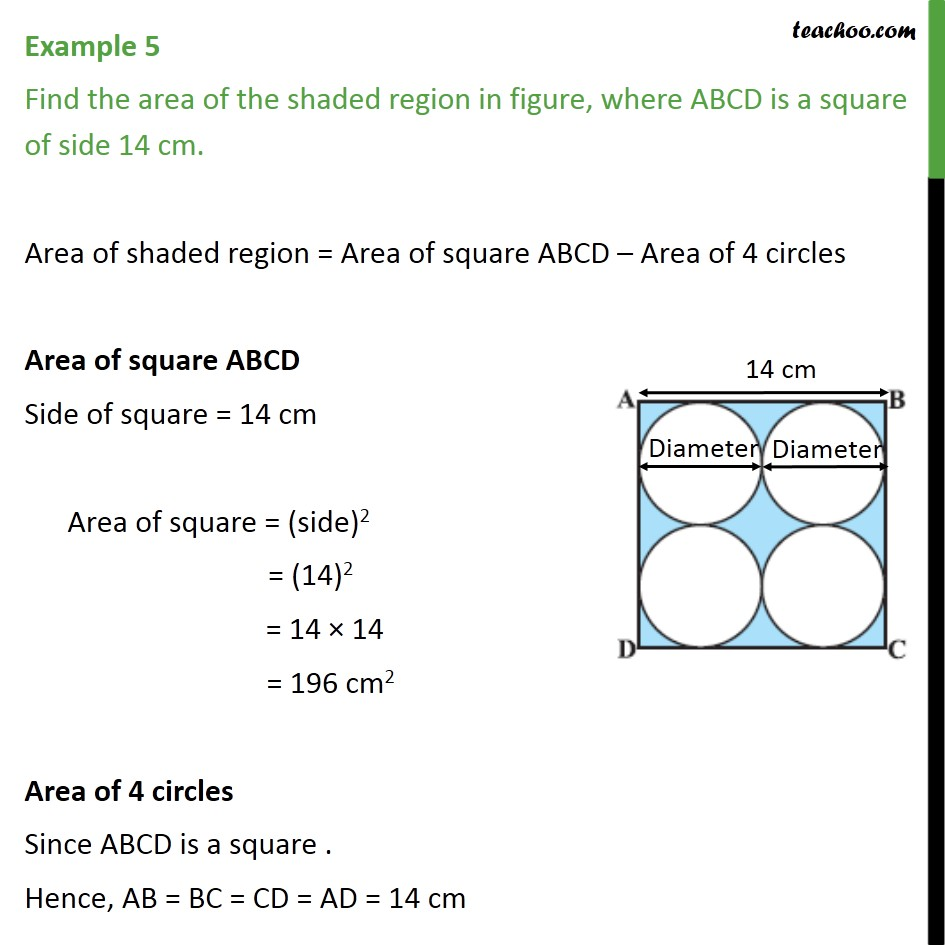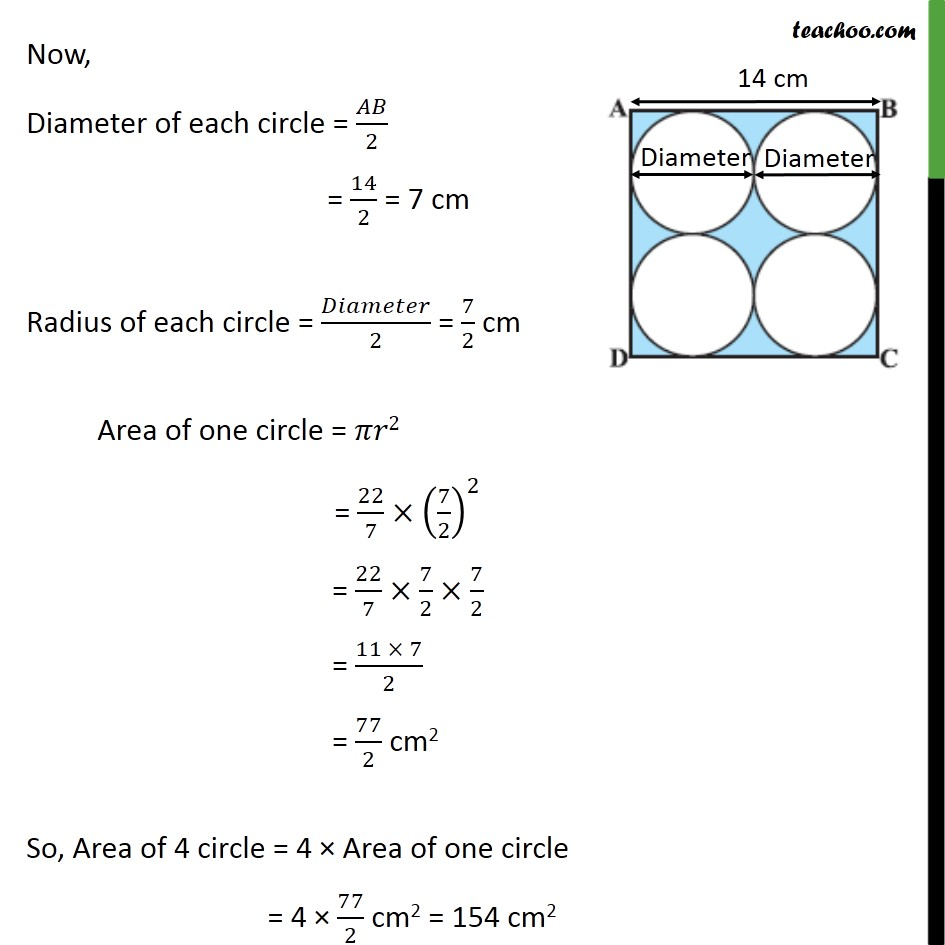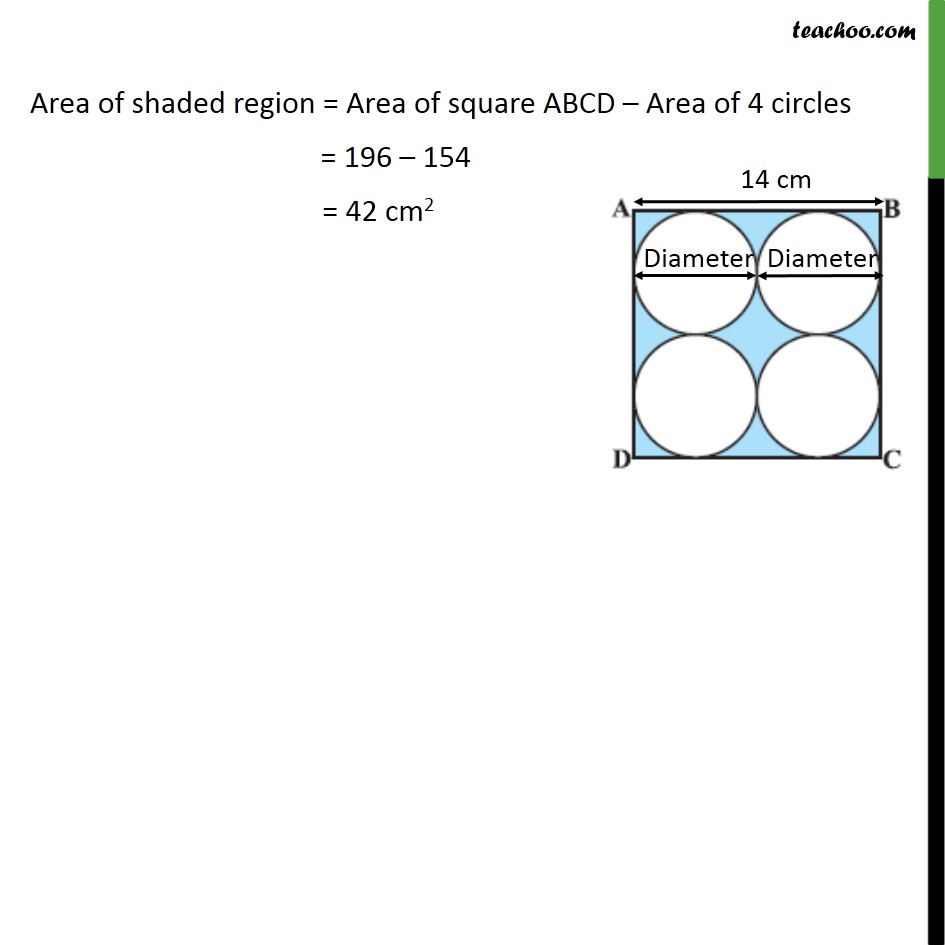Area of combination of figures : circle based

Chapter 11 Class 10 Areas related to Circles
Concept wiseLearn in your speed, with individual attention - Teachoo Maths 1-on-1 Class

### Transcript

Question 3 Find the area of the shaded region in figure, where ABCD is a square of side 14 cm. Area of shaded region = Area of square ABCD – Area of 4 circles Area of square ABCD Side of square = 14 cm Area of square = (side)2 = (14)2 = 14 × 14 = 196 cm2 Area of 4 circles Since ABCD is a square . Hence, AB = BC = CD = AD = 14 cm Now, Diameter of each circle = 𝐴𝐵/2 = 14/2 = 7 cm Radius of each circle = 𝐷𝑖𝑎𝑚𝑒𝑡𝑒𝑟/2 = 7/2 cm Area of one circle = 𝜋𝑟2 = 22/7×(7/2)^2 = 22/7×7/2×7/2 = (11 × 7)/2 = 77/2 cm2 So, Area of 4 circle = 4 × Area of one circle = 4 × 77/2 cm2 = 154 cm2 Area of shaded region = Area of square ABCD – Area of 4 circles = 196 – 154 = 42 cm2